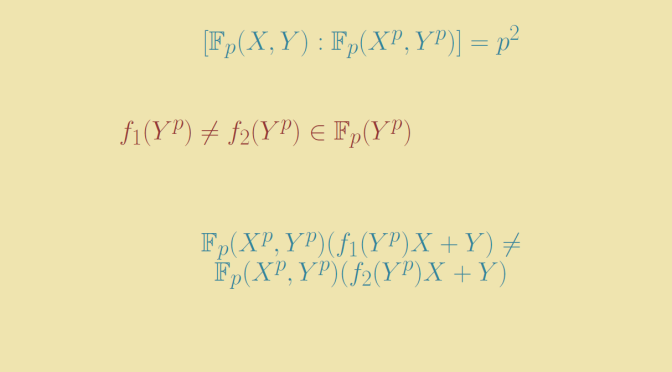# A finite extension that contains infinitely many subfields

Let’s consider $$K/k$$ a finite field extension of degree $$n$$. The following theorem holds.

Theorem: the following conditions are equivalent:

1. The extension contains a primitive element.
2. The number of intermediate fields between $$k$$ and $$K$$ is finite.

Our aim here is to describe a finite field extension having infinitely many subfields. Considering the theorem above, we have to look at an extension without a primitive element.

### The extension $$\mathbb F_p(X,Y) / \mathbb F_p(X^p,Y^p)$$ is finite

For $$p$$ prime, $$\mathbb F_p$$ denotes the finite field with $$p$$ elements. $$\mathbb F_p(X,Y)$$ is the algebraic fraction field of two variables over the field $$\mathbb F_p$$. $$\mathbb F_p(X^p,Y^p)$$ is the subfield of $$\mathbb F_p(X,Y)$$ generated by the elements $$X^p,Y^p$$.

The degree of $$\mathbb F_p(X,Y) / \mathbb F_p(X^p,Y^p)$$ is equal to $$p^2$$. To prove it, one can see on one side that $$\{X^iY^j \ : \ 0 \le i,j \le p-1\}$$ are independent elements over $$\mathbb F_p(X^p,Y^p)$$ which proves that $$[\mathbb F_p(X,Y) : \mathbb F_p(X^p,Y^p)] \ge p^2$$. On the other side a polynomial $$P(X,Y) \in \mathbb F_p(X,Y)$$ can be written as a linear combination $\sum_{0 \le i,j \le p-1} \lambda_{ij} X^iY^j$ where $$\lambda_{ij} \in \mathbb F_p[X^p,Y^p]$$. And an element $$\frac{P}{Q} \in \mathbb F_p(X,Y)$$ is equal to $$\frac{PQ^{p-1}}{Q^p}$$ while $${Q^p}$$ belongs to $$\mathbb F_p[X^p,Y^p]$$ as can be proved using Frobenius endomorphism. Finally, $$\frac{P}{Q}$$ can be written as linear combination $$\displaystyle \sum_{0 \le i,j \le p-1} \alpha_{ij} X^iY^j$$ where $$\alpha_{ij} \in \mathbb F_p(X^p,Y^p)$$ and we can conclude to $$[\mathbb F_p(X,Y) : \mathbb F_p(X^p,Y^p)] = p^2$$.

### The extension $$\mathbb F_p(X,Y) / \mathbb F_p(X^p,Y^p)$$ is not simple

For $$\theta \in \mathbb F_p(X,Y)$$ we have $$\theta^p \in \mathbb F_p(X^p,Y^p)$$ using again Frobenius endomorphism. Hence $$[\mathbb F_p(X^p,Y^p)(\theta) : \mathbb F_p(X^p,Y^p)] \le p$$ proving that $$\mathbb F_p(X,Y) / \mathbb F_p(X^p,Y^p)$$ cannot have a generating element.

The theorem above allows us to conclude that $$\mathbb F_p(X,Y) / \mathbb F_p(X^p,Y^p)$$ has infinitely many subfields.

### Infinitely many subfields of $$\mathbb F_p(X,Y) / \mathbb F_p(X^p,Y^p)$$

For $$f(Y^p) \in \mathbb F_p(Y^p)$$, the field: $K=\mathbb F_p(X^p,Y^p)(f(Y^p)X+Y)$ is a nontrivial extension of $$\mathbb F_p(X^p,Y^p)$$ and we can get that way infinitely many subfields between $$\mathbb F_p(X^p,Y^p)$$ and $$\mathbb F_p(X,Y)$$. Because for $$f_1(Y^p) \neq f_2(Y^p) \in \mathbb F_p(Y^p)$$ if $$\mathbb F_p(X^p,Y^p)(r)=\mathbb F_p(X^p,Y^p)(s)$$ with $$r=f_1(Y^p)X+Y$$ and $$s=f_2(Y^p)X+Y$$, we get $$X = (f_1(Y^p) – f_2(Y^p))^{-1}(r-s) \in \mathbb F_p(X^p,Y^p)(r)$$, then $$Y \in \mathbb F_p(X^p,Y^p)(r)$$ and $$\mathbb F_p(X,Y)$$ would be finitely generated by $$r$$. Hence for $$f_1 \neq f_2$$ the extensions $$\mathbb F_p(X^p,Y^p)(f_1(Y^p)X+Y)$$ and $$\mathbb F_p(X^p,Y^p)(f_2(Y^p)X+Y)$$ are different.